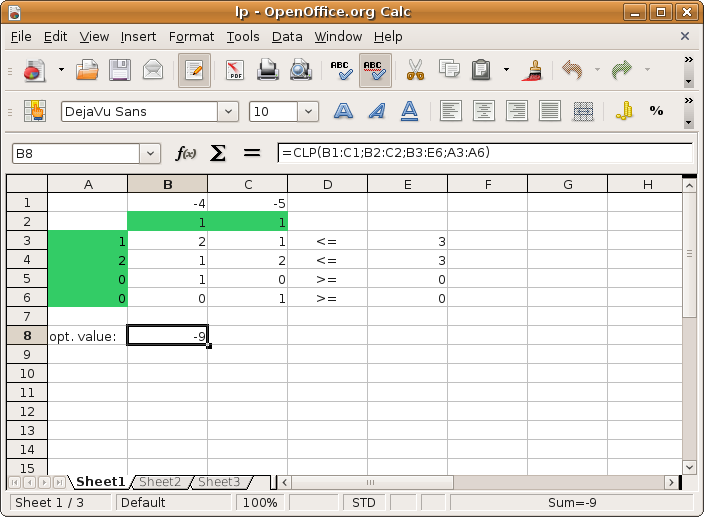# OpenOffice plugin

The OpenOfficece.org plugin provides a spreadsheet interface to the basic optimization solvers in CVXOPT.

As a simple example, the following linear programming problem,

$\begin{split}\begin{array}[t]{ll} \mbox{minimize} & -4x_1 - 5x_2 \\ \mbox{subject to} & 2x_1 + x_2 \leq 3 \\ & x_1 + 2x_2 \leq 3 \\ & x_1 \geq 0, \quad x_2 \geq 0. \end{array}\end{split}$

can be solved using a spreadsheet as shown in the figure below,